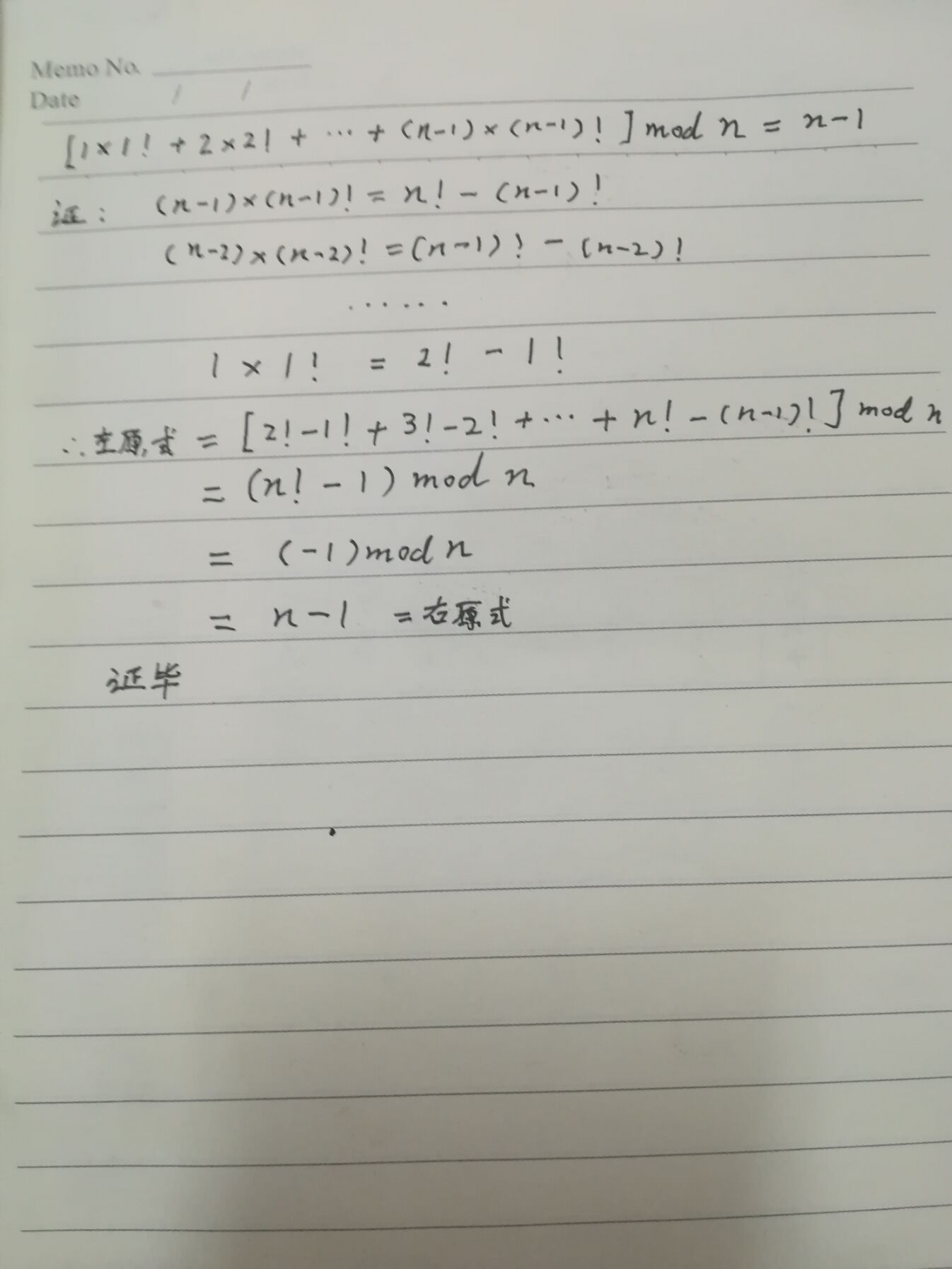PS：n! = 1 * 2 * …… * nAlice, a student of grade 66, is thinking about an Olympian Math problem, but she feels so despair that she cries. And her classmate, Bob, has no idea about the problem. Thus he wants you to help him. The problem is:

We denote k!k!:

k! = 1 \times 2 \times \cdots \times (k - 1) \times kk!=1×2×⋯×(k−1)×k

We denote SS:

S = 1 \times 1! + 2 \times 2! + \cdots +S=1×1!+2×2!+⋯+
(n - 1) \times (n-1)!(n−1)×(n−1)!

Then SS module nn is ____

You are given an integer nn.

You have to calculate SS modulo nn.

### Input

The first line contains an integer T(T \le 1000)T(T≤1000), denoting the number of test cases.

For each test case, there is a line which has an integer nn.

It is guaranteed that 2 \le n\le 10^{18}2≤n≤1018.

### Output

For each test case, print an integer SS modulo nn.

### Hint

The first test is: S = 1\times 1!= 1S=1×1!=1, and 11modulo 22 is 11.

The second test is: S = 1\times 1!+2 \times 2!= 5S=1×1!+2×2!=5, and 55 modulo 33 is 22.

#### 题目来源

ACM-ICPC 2018 南京赛区网络预赛